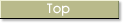###Section 1 Layout of Figures

 Section One has ten parts for the ten figures shown in the initial pages of this book: 1. Figure T. 2. Figure A. 3. Figure S.  4. Figure V. 5. Figure X 6. Figure of Theology 7. Figure of Philosophy 8. Figure of Law 9. Elemental Figure 10. Common Figure T. stands for the figure of Principles,  A. for the figure of God,  S. for the figure of the Soul,  V. for the figure of Virtues and Vices  X. for the figure of Opposites.Site contents